Finding General Solutions

Chapter 3 Class 11 Trigonometric Functions
Concept wise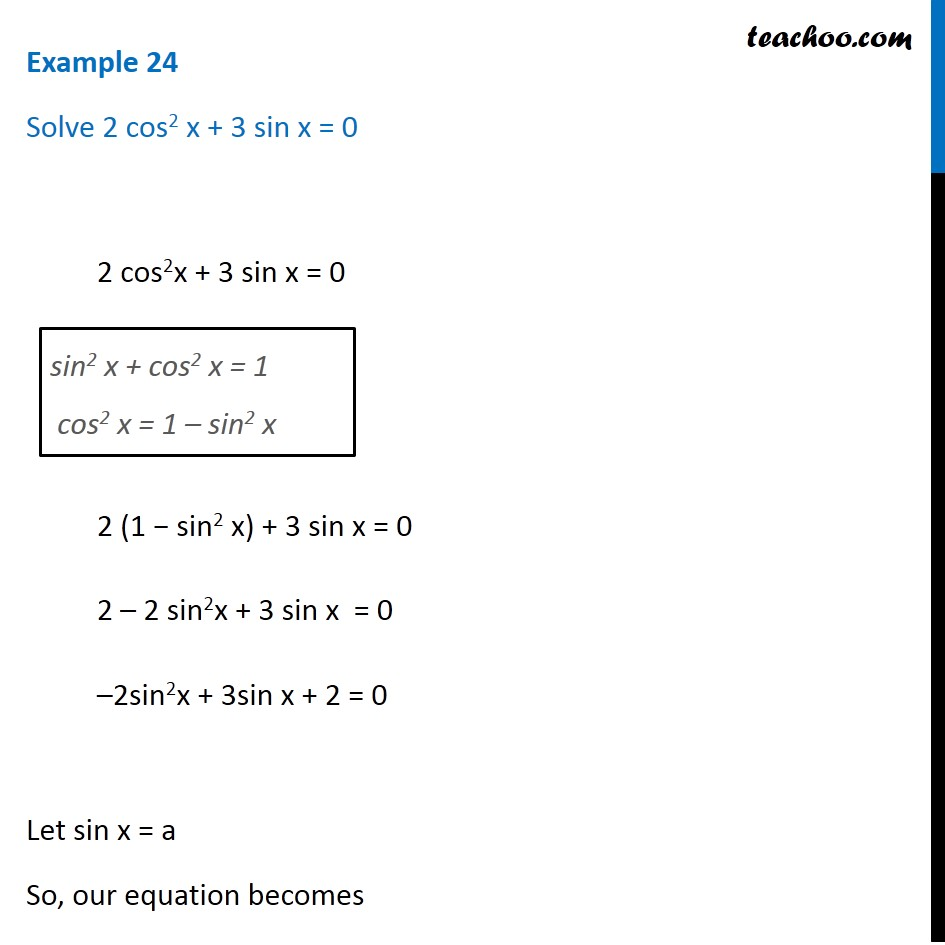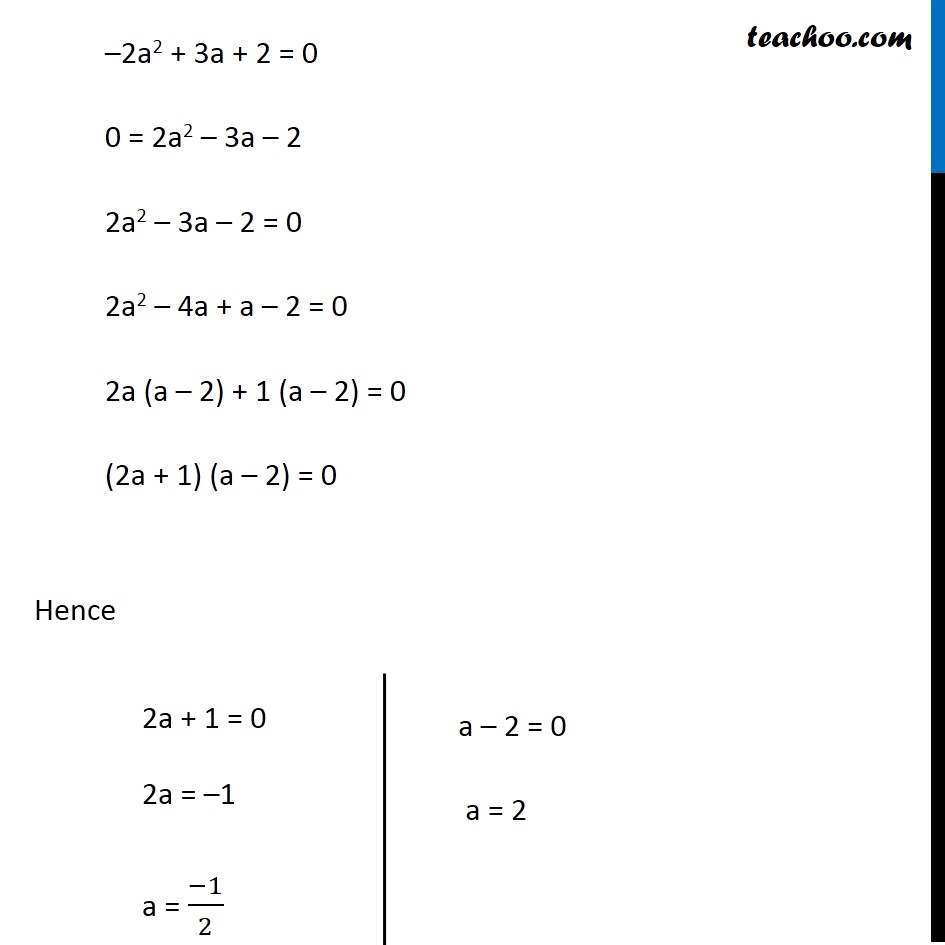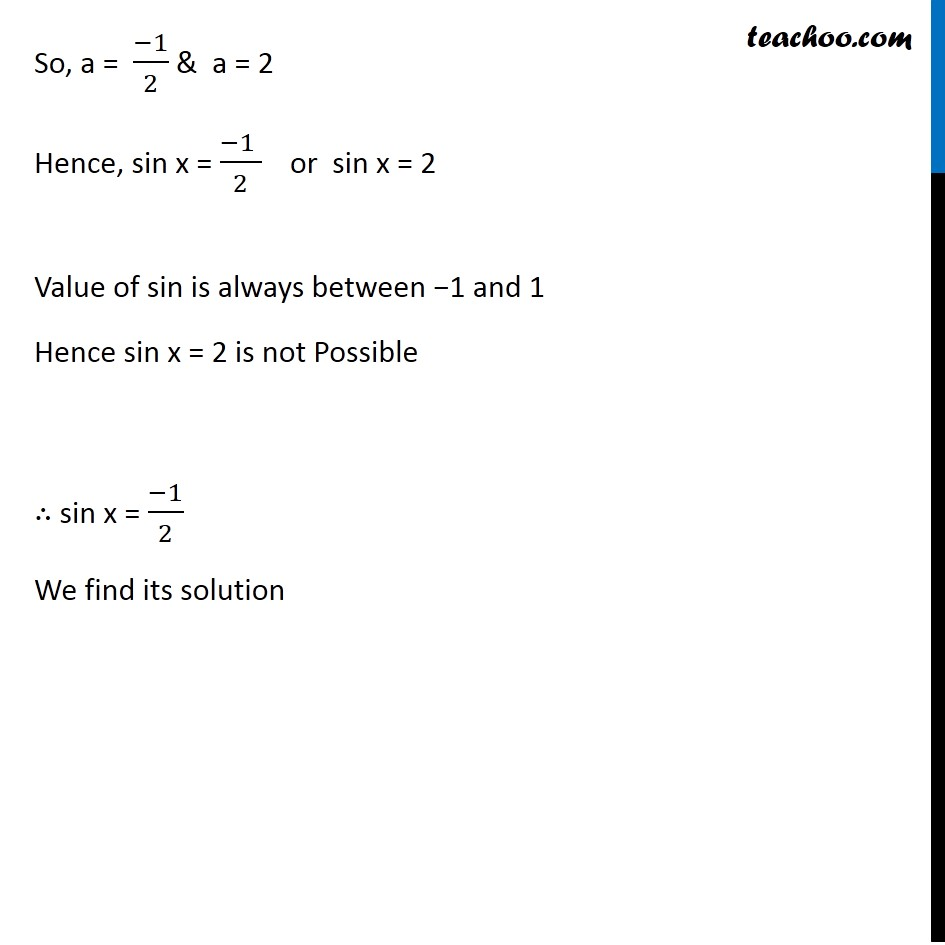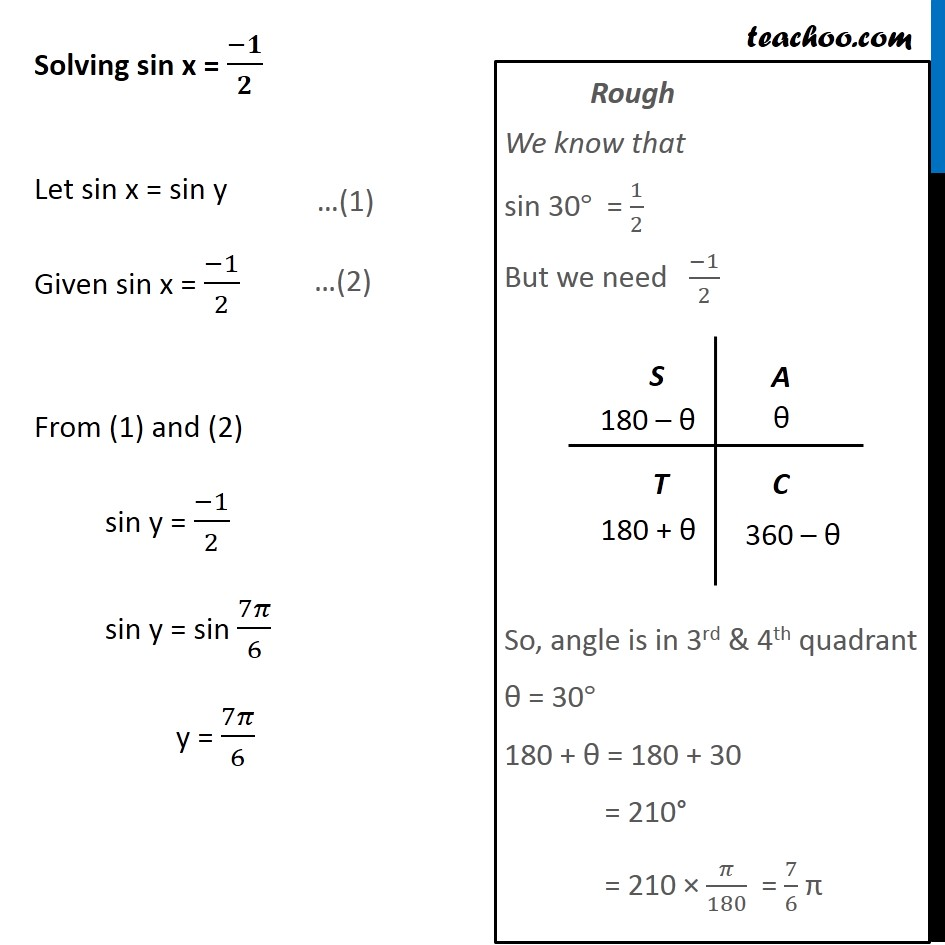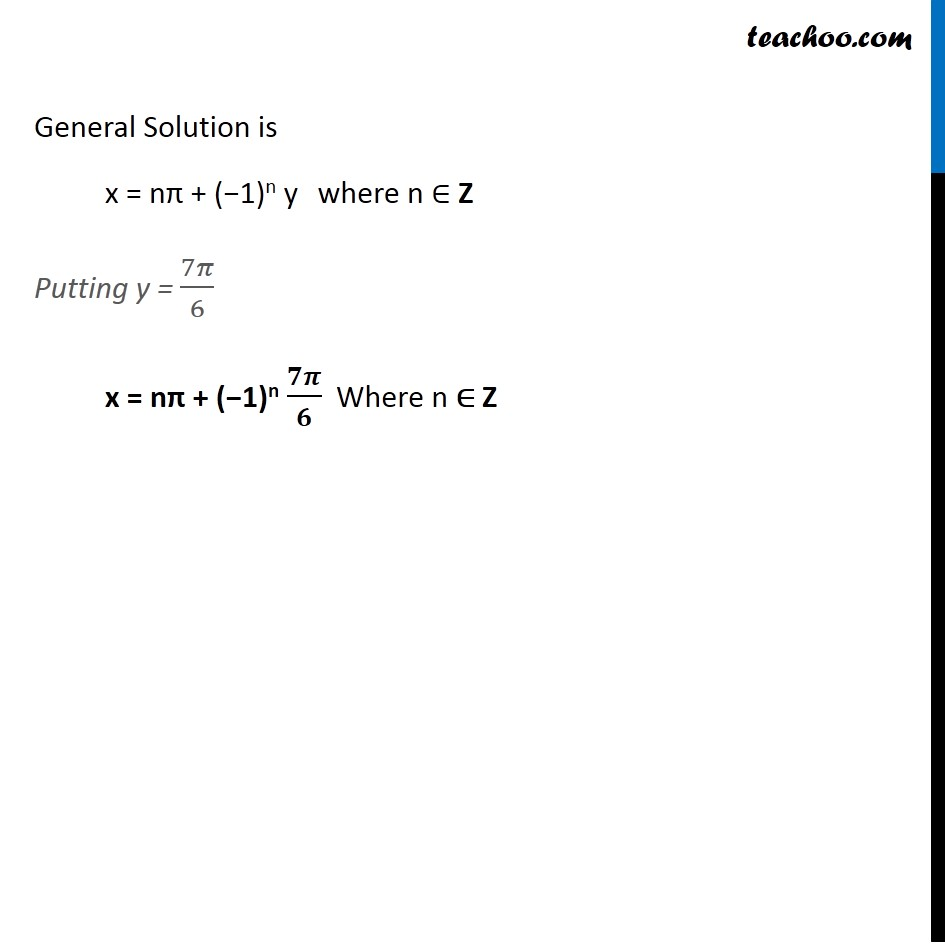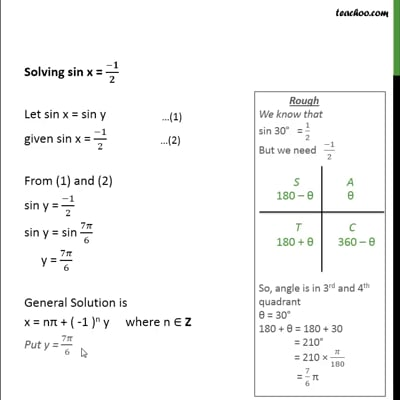This video is only available for Teachoo black users

Introducing your new favourite teacher - Teachoo Black, at only ₹83 per month

### Transcript

Example 24 Solve 2 cos2 x + 3 sin x = 0 2 cos2x + 3 sin x = 0 2 (1 − sin2 x) + 3 sin x = 0 2 – 2 sin2x + 3 sin x = 0 –2sin2x + 3sin x + 2 = 0 Let sin x = a So, our equation becomes sin2 x + cos2 x = 1 cos2 x = 1 – sin2 x –2a2 + 3a + 2 = 0 0 = 2a2 – 3a – 2 2a2 – 3a – 2 = 0 2a2 – 4a + a – 2 = 0 2a (a – 2) + 1 (a – 2) = 0 (2a + 1) (a – 2) = 0 Hence 2a + 1 = 0 2a = –1 a = (−1)/2 a – 2 = 0 a = 2 So, a = (−1)/2 & a = 2 Hence, sin x = (−1 )/2 or sin x = 2 Value of sin is always between −1 and 1 Hence sin x = 2 is not Possible ∴ sin x = (−1)/2 We find its solution Solving sin x = (−𝟏)/𝟐 Let sin x = sin y Given sin x = (−1)/2 From (1) and (2) sin y = (−1)/2 sin y = sin 7𝜋/6 y = 7𝜋/6 Rough We know that sin 30° = 1/2 But we need (−1)/2 So, angle is in 3rd & 4th quadrant θ = 30° 180 + θ = 180 + 30 = 210° = 210 × 𝜋/180 = 7/6 π Rough We know that sin 30° = 1/2 But we need (−1)/2 So, angle is in 3rd & 4th quadrant θ = 30° 180 + θ = 180 + 30 = 210° = 210 × 𝜋/180 = 7/6 π General Solution is x = nπ + (−1)n y where n ∈ Z Putting y = 7𝜋/6 x = nπ + (−1)n 𝟕𝝅/𝟔 Where n ∈ Z